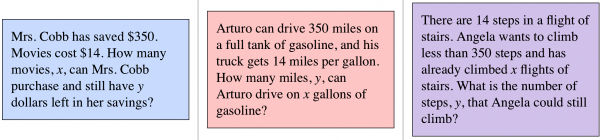# Let's Get Started

Let's practice how to graph one-variable and two-variable inequalities and how to determine the symbolic representation of an inequality given a verbal statement.

TEKS Standards and Student Expectations

A(2) Linear functions, equations, and inequalities. The student applies the mathematical process standards when using properties of linear functions to write and represent in multiple ways, with and without technology, linear equations, inequalities, and systems of equations. The student is expected to:

A(2)(C) write linear equations in two variables given a table of values, a graph, and a verbal description

A(2)(H) write linear inequalities in two variables given a table of values, a graph, and a verbal description

Resource Objective(s)

Given a problem situation in verbal form, students will write an inequality that can be used to represent the situation.

Essential Questions

What words mean less than/less than or equal to?

What words mean greater than/greater than or equal to?

What are the four different inequality symbols and what do they represent?

Vocabulary

# Introduction

You may have used algebra to write equations that represent real-world problem situations. Sometimes we need to use algebra to describe situations that may call for a range of solutions instead of one particular solution. When that is the case we can use an inequality to describe the situation instead of an equation.

Consider the number line shown. Remember that on a number line, values increase from left to right.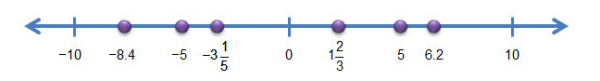We use four inequality symbols to represent unequal relationships.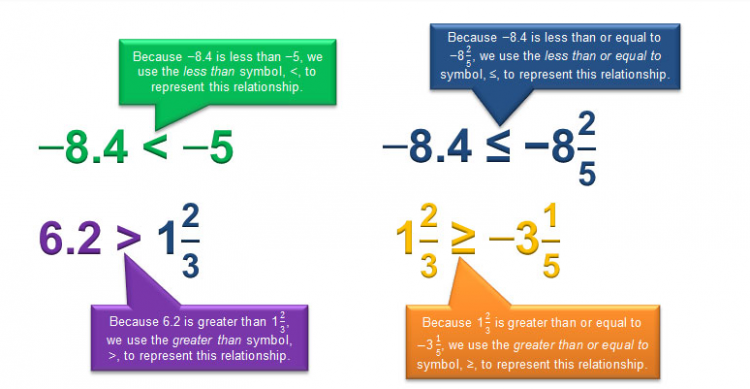These four inequality symbols can be used to represent relationships.

# Clue Words and Inequality Symbols

Clue words may help you determine when you need an inequality instead of an equation. Click on the inequality symbol to see some of clue words that suggest particular inequality conditions.

# Determining “Less Than,” “Greater Than,” or “Equal”

One of the biggest challenges you may face when writing inequalities is determining which inequality symbol to use. It is important to underline and/or highlight clue words within your problem to help you determine the symbol needed. Use the chart you copied in the last activity to help.

• How can you tell if a situation involves "less than"?
• How can you tell if a situation involves "greater than"?
• How can you distinguish between situations involving "less than" and situations involving "less than or equal to"?
• How can you distinguish between situations involving "greater than" and situations involving "greater than or equal to"?

Practice

Write an inequality representing each of the situations below.

1. Victor plans to walk at least 30 miles in a charity walk-a-thon. He has walked 12 miles so far. Write an inequality to determine how many more miles Victor must walk in order to meet his goal.

2. 12 more than a number is at most 30. Write an inequality to determine the number.

3. Roxanne spent $30 on a shirt at the mall and had no more than$12 left over. Write an inequality to determine the amount of money Roxanne took to the mall.

4. Yvonne earned \$12 per hour babysitting last weekend. She worked no less than 30 hours. Write an inequality to determine the amount of money Yvonne earned last weekend.

# Writing Inequalities from Problem Situations

You may have studied ways to represent a linear function using the starting point and a rate of change. You may have called this form of a linear function slope-intercept form.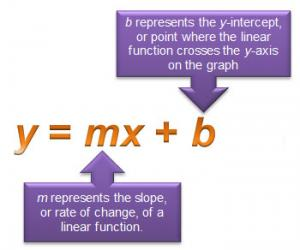You also examined situations that could result in equations written in standard form. Standard form of a linear equation is Ax + By = C. In this representation, A, B, and C are constants that show the relationship between two variables, x and y.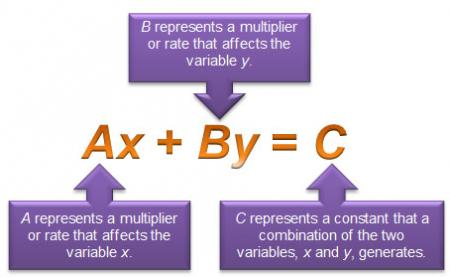# Creating Problem Situations from Inequalities

We know you can write inequalities to describe a given situation, but you can also apply that skill to do the opposite—write a situation to match a given inequality.

Consider the following inequality: y 14x.

This inequality could describe any of the following situations.